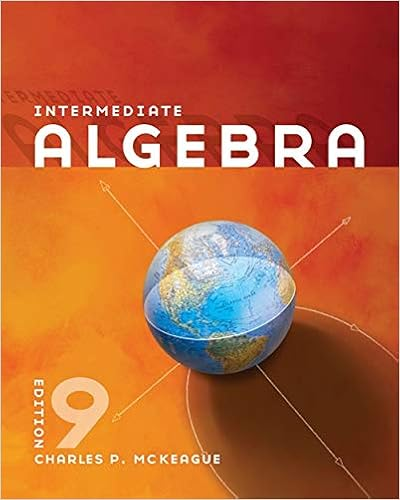# Week+3-Chapter+3+In+class+Activities+A.docx - Math 040...

• Homework Help
• 3
• 50% (2) 1 out of 2 people found this document helpful

This preview shows page 1 - 3 out of 3 pages.

##### We have textbook solutions for you!
The document you are viewing contains questions related to this textbook.The document you are viewing contains questions related to this textbook.
Chapter 1 / Exercise 61
Intermediate Algebra
MckeagueExpert Verified
Math 040 : Re-Entry Mathematics Franklin UniversityWeek 3 Chapter 3 In class activitiesName: _Chandra Vargovich__1.Identify like terms: a.8b, 17y, -22, 14y, 4xy, 17, -9xy, -9h _17y, 14y and -22, 17 and 4xy, -9xy2.Apply the associative property, the commutative property or the distributive property to the given expression and rewrite or simplify the expression.a.a(-4) __________________________________________________________ b.7 + (5 + p) ______________________________________________________c.3(6x) __________________________________________________________d.-3(-5q – 2s – 3t) __________________________________________________3.Combine like terms by adding, subtracting or clearing parentheses.a.14a – 5b + 3a – b – 3 ___________________________________________ b.– 3( 2x + 1) – 13 _ _________________________________________________c.– 5 – 11p + 23p – p + 4 __________________________________________d.–( 8w + 5) + 3(w – 15) ___________________________________________4.Identify as an expression or equation.a.8x – 9 – 7 _________________________________________________ b.2( x – 4) – x = 1 _____________________________________________ c.24 – 5x + 12 + x ______________________________________________ 5.Determine whether the given number is a solution to the equation.a.3y – 2 = 4; 2 _______________________b.39 = – 7p + 4; 5 _____________________c.–z + 8 = 20; 12 _____________________6.Solve the equations:a.– 4 = y + 18d. v10= – 4 b.-8k = 56e. 3(r – 2) – 2r = – 2 + 6 1050315
##### We have textbook solutions for you!
The document you are viewing contains questions related to this textbook.The document you are viewing contains questions related to this textbook.
Chapter 1 / Exercise 61
Intermediate Algebra
MckeagueExpert Verified
Math 040 : Re-Entry Mathematics Franklin Universityc.11 – 7x + 8x = – 4 – 27.Solve the equations:a.–3k + 14 = – 4 +3k d. 6(w +2) = 20 + 2wb.-3x + x 8 = -2(6 – x) e. 6h – 2(h + 6) = 10hc.
•••